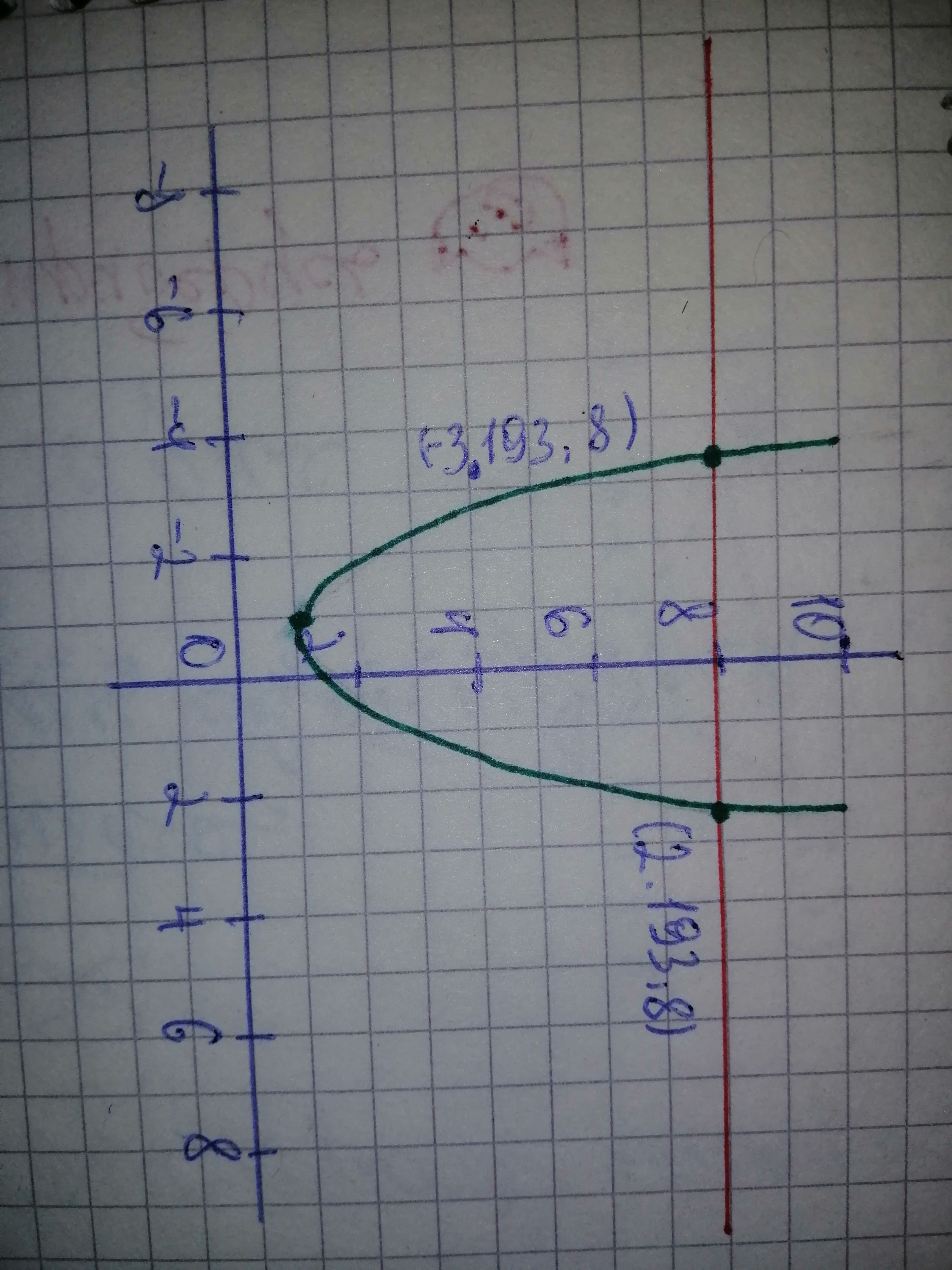# Equation: 8 divided by displaystyle{left({x}^{2}+{x}+{1}right)}={1} Use crossing graphs method to solve the above equation. There are 2 solutions. RouBergen 2021-02-19 Answered
Equation: 8 divided by $\left({x}^{2}+x+1\right)=1$
Use crossing graphs method to solve the above equation. There are 2 solutions. Round answers to 2 decimals. Provide smaller and larger value:
$x=\left(smal\le rvalue\right)x=\left(lar\ge rvalue\right)$
You can still ask an expert for help

• Questions are typically answered in as fast as 30 minutes

Solve your problem for the price of one coffee

• Math expert for every subject
• Pay only if we can solve ithosentak

Step 1
8 divided by $\left({x}^{2}+x+1\right)=1$
This means,
$8\text{/}\left({x}^{2}+x+1\right)=1$
Hence, $8={x}^{2}+x+1$
Step 2
Let's plot two graphs:
$y=8$
and $y={x}^{2}+x+1$There are two solutions (the points of intersection):
(smaller value)
$x=2.19$ ( larger value)